Solving algebraic equations rationally

Aim: Finding and mastering new rational ideas for solving algebraic equations.

Example 1. Solve the equation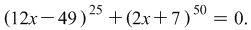Solution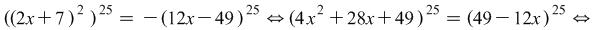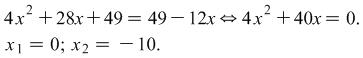Example 2. Solve the equation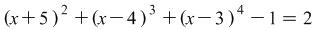.

Solution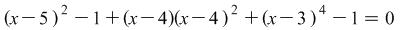i.e. the number 2 is represented as the sum of 1+1.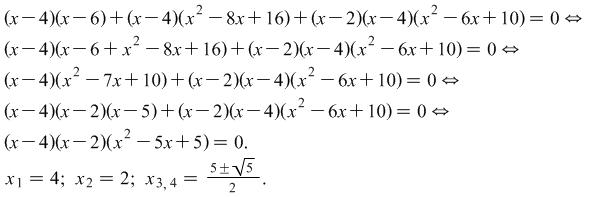Example 3. Solve the equation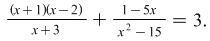Solution If we use the standard method - eliminating the denominator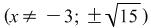and doing subsequent transformations we get the equation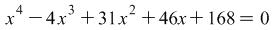. We find the divisors of the free term, they are 32 numbers and through direct verification the roots of the equation are found.

The problem can be solved more rationally if the person solving it guesses that here the number 3 is suitable to be represented as 4-1.

Then the given equation can be presented in the form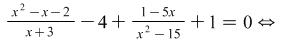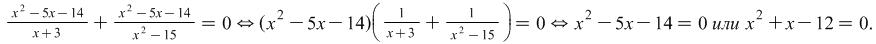The roots of the given equation are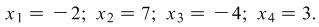# For individual work

Problem  1. Solve the equation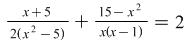.

Answer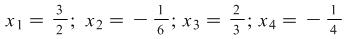.

Problem  2. Solve the equation.

Answer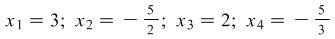.

By Rumyana Mavrova, Plovdiv university, rummav@pu.acad.bg